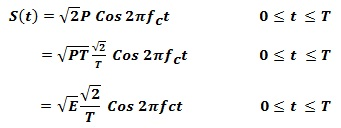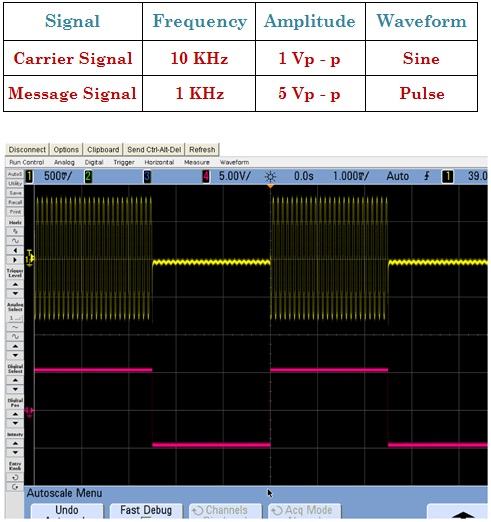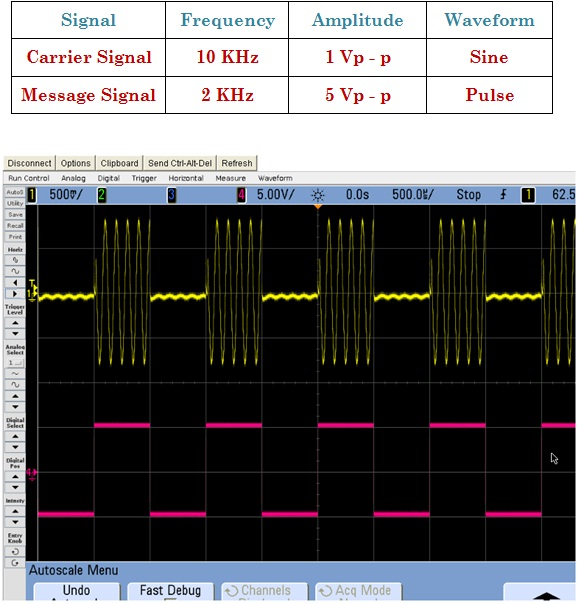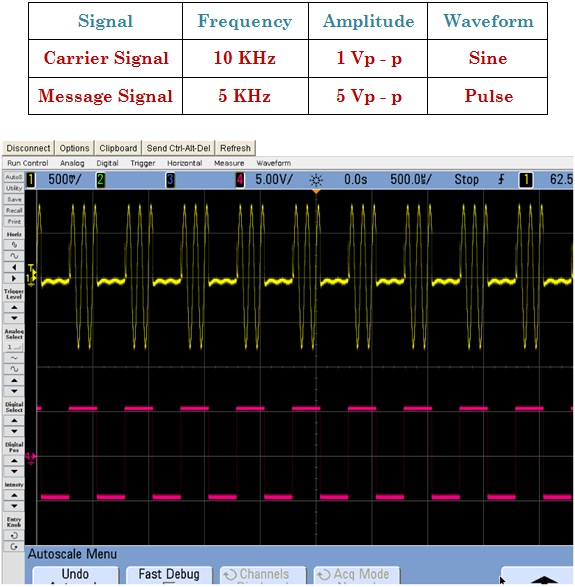Amplitude Shift Keying

Calculations & Observations

A binary amplitude shift keying signal can be defined byWhere A is a binary constant, m (t) = 1 or 0, fc is the carrier frequency, and T is the bit duration. It has a power P = A2 / 2, so that A = √2P.

Thus equation can be written asWhere E = PT is the energy contained in a bit duration.